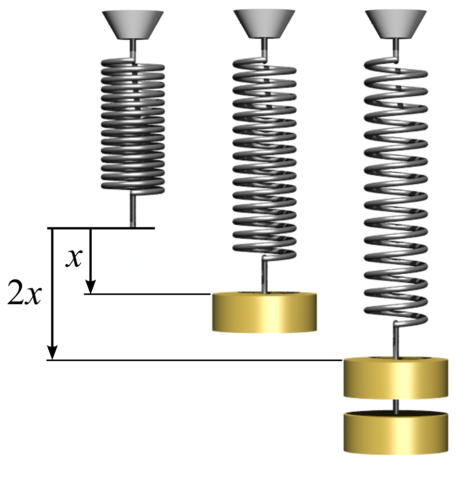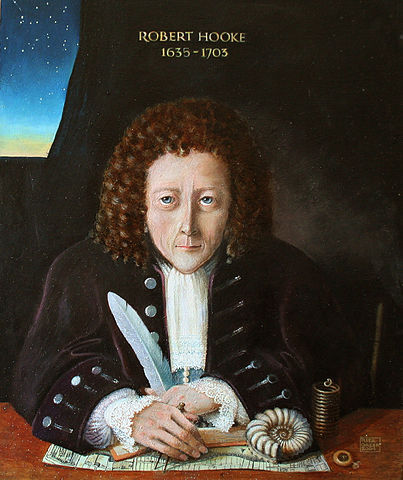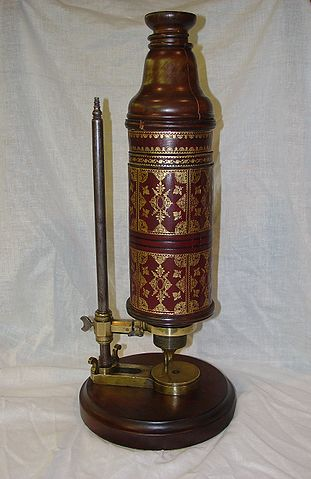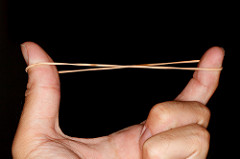November 26, 2018

## To become a Scientist

Someone who does Science

Science is not only making experiments

Science is the process of creating knowledge

We search for truth with the Scientific Method

## Scientific Method

• We observe the nature and find patterns
• We create models that can explain the patterns
• We make experiments to test if the models are valid

This way we avoid fooling ourselves

## Scientist look for the truth

• Does smoking causes cancer?
• Does eating sugar makes you fat?
• Does your cellphone produce brain cancer?
• Will an expensive medicine cure your sickness?

The society expect from us, the scientists, to answer these questions with the truth

## Even in everyday jobs

Tomorrow you may work in a blood bank. Is the blood safe?

Or in a food factory. Is the food safe? Is it GMO?

Or in a University. Is this pharmaceutical company telling the truth?

Or you do a paternity test. Is this person the real father?

## Truth is essential for scientists

• Sometimes you get a wrong result
• Sometimes your model is incomplete
• Most models are incomplete, and we are always updating them

You can be wrong, but you cannot lie

## Some key parts of the Scientific Method

• Models are tested with experiments

• To be valid, experiments must be replicable

• That is, other people doing the same experiment must get the same result
• There may be some variation between experiments

• You must declare what is your margin of error
• Every measurement has a margin of error

## Coils• Coils and rubber bands have a natural size
• If you apply a force to them, they expand
• What is the relation between the expansion and the force?
• We can put different weights and use gravity force

## Results

n_marbles length repetition
0 78.00 1
1 82.61 1
2 85.85 1
3 90.26 1
4 95.05 1
0 79.21 2
2 85.55 2
3 90.06 2
4 94.35 2
(some data o mitted fr om the table)## Best-fit line

When data seems to be in a straight line, we can find that line

The best-fitting line is found using a linear model

model <- lm(n_marbles ~ length, data=rubber)
model
Call:
lm(formula = n_marbles ~ length, data = rubber)

Coefficients:
(Intercept)       length
-14.5354       0.1915  

## What are the coefficients?

Remember that straight lines can be represented by the formula $\text{n_marbles}=A+B\cdot \text{length}$ The coefficient $$A$$ is the value where the line intercepts the vertical axis

The coefficient $$B$$ is how much length goes up when n_marbles increases. This is called slope

In our case $$A$$ and $$B$$ are

(Intercept)      length
-14.5353768   0.1914521 

## Robert Hooke said it firstRobert Hooke (1635–1703) was an English natural philosopher, architect and polymath.

In 1660, Hooke discovered the law of elasticity which describes the linear variation of tension with extension

“The extension is proportional to the force”

## Robert HookeNatural philosophy was the study of nature and the physical universe that was dominant before the development of modern science

Polymath (from Greek “having learned much”) is a person whose expertise spans a significant number of different subject areas

Biologist. Hooke used the microscope and was the fists to use the term cell for describing biological organisms.

## How do we model a coil?

The essence of the coil is:

• It has a natural length $$L$$
• If we change the length by $$x$$, it pulls with a force $\mathrm{force}(x)= K \cdot (L-x)$## Physical interpretation of the linear model

The formula from Hooke’s Law is $\text{force}=K\cdot(L-\text{length})$ Since force is the weight of the marbles, we can write $-m g\cdot\text{n_marbles}=K\cdot(L-\text{length})$ which can be re-written as $\text{n_marbles}=\frac{K}{m g}\cdot\text{length} - \frac{K}{m g}\cdot L$

## Physical interpretation of coef(model)

Comparing the formulas we can see that $\texttt{coef(model)}=\frac{K}{m g}\quad\text{thus}\quad K=\texttt{coef(model)}\cdot m\cdot g$ If the mass of each ball is 20gr, we can find $$K$$ as

coef(model) * 20 * 9.8
  length
37.52461 

This is the elasticity constant. The units are dyne/cm

## Natural length of the coil

We also see that $\texttt{coef(model)}=-\frac{K}{m g}L = -\texttt{coef(model)}\cdot L$ Therefore $L=-\frac{\texttt{coef(model)}}{\texttt{coef(model)}}$

## Natural length of the coil

When there are no balls, the natural length of the coil is $$L$$

This value is hard to measure directly

But, using the formula from the regression, we have

-coef(model)/coef(model)
(Intercept)
75.92175 

## Can we replicate your experiments?

I cleaned up all the files

There are two that I could not recover

The rest are either “coins” or “marbles”

## Coins

A B K L
-32.640000 4.3200000 347.15520 7.555556
-22.631714 3.1202046 250.73964 7.253279
-14.514493 1.8840580 151.40290 7.703846
-13.590202 1.9517885 156.84572 6.962948
-12.349076 1.6837782 135.30842 7.334146
-11.000000 1.2857143 103.32000 8.555556
-9.621145 2.9074890 233.64581 3.309091
-8.728814 1.1525424 92.61831 7.573529
-5.058176 0.5554427 44.63538 9.106567

## Plot Coins## Marbles

A B K L
-18.305520 2.2721438 445.34018 8.056497
-11.285714 1.4285714 280.00000 7.900000
-10.039956 1.2005156 235.30105 8.363037
-9.366083 1.9008064 372.55805 4.927426
-5.048315 0.5550562 108.79101 9.095142
-5.048315 0.5550562 108.79101 9.095142
-4.986521 0.5518820 108.16887 9.035484
-3.278303 0.0643182 12.60636 50.970104

## Plot Marbles## How cells grow

We want to know the number of cells every day, which we represent with the vector ncell

Each element of the vector is the number of cells in day t

We start with an initial number of cells, that we call initial

Each day, the number of cells increases by a factor rate

## Recurrence

The number of cells on day t is ncell[t]

Each day the number of cell is multiplied by rate

Therefore ncell[t] = rate * ncell[t-1]

This is a recursive formula

Can you write an explicit formula? (non-recursive)

## Formula

The solution of the recurrence is

ncell[t] = initial * rate^t

In R we can do this easily when t is a vector

t <- seq(from=start, to=end, by=step)
ncell <- initial * rate ^ t

## Graphic

t <- 1:20
initial <- 20
rate <- 2
ncells <- initial * rate^t
ncells
        40       80      160      320      640     1280     2560     5120    10240
    20480    40960    81920   163840   327680   655360  1310720  2621440  5242880
 10485760 20971520

## Graphic

plot(t, ncells)We cannot see what happens when values are small

## Logarithmic scale (“semi-log”)

plot(log(ncells) ~ t)We can see better using a logarithmic vertical scale

## Why?

We have many cases when the values increase (or decrease) with a factor

For example, the cost of DNA sequencing

In general if the relation is $y = I\cdot R^x$ we can get a better picture applying logarithms $\log(y) = \log(I\cdot R^x) = \log(I) + x \log(R)$

## Other cases

Sometimes the formula is different. For example the area of a circle is $a=\pi r^2$ and the volume of a sphere is $v=\frac{4}{3}\pi r^3$

## General case

In general you can have $y= A x^B$ then, using logarithms, you have $\log(y)=\log(A x^B)=\log(A) + B\log(x)$ Now we need also $$\log(x)$$

## Log-log scale

par(mfrow=c(1,2))
plot(t, 2*t^3)
plot(t, 2*t^3, log="xy")When the logarithmic scale shows a straight line, we can use a linear model

We have to be careful to use log and exp in the correct place

## Example

Let’s consider this case

example <- data.frame(t, vol=2*t^3)
head(example)
  t vol
1 1   2
2 2  16
3 3  54
4 4 128
5 5 250
6 6 432

## Plot Using logarithm

par(mfrow=c(1,2))
plot(vol~t, data=example)
plot(log(vol)~t, data=example)Log-log scale looks straight

## Model

model <- lm(log(vol)~log(t), data=example)
model
Call:
lm(formula = log(vol) ~ log(t), data = example)

Coefficients:
(Intercept)       log(t)
0.6931       3.0000  
exp(coef(model))
(Intercept)
2